# ISEE Middle Level Math : Data Analysis

## Example Questions

### Example Question #51 : Data Analysis

Find the range for this set of numbers: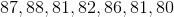Possible Answers: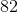Correct answer:Explanation:

First, order the numbers from least to greatest: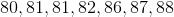Then, subtract the smallest number from the largest: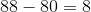Answer:### Example Question #52 : Data Analysis

Find the range in this set of numbers: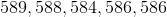Possible Answers:Correct answer:Explanation:

First order the numbers from least to greatest: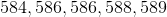Then, subtract the smallest number from the largest: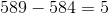Answer:### Example Question #52 : Data Analysis And Probability

Find the range in this set of numbers: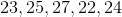Possible Answers:Correct answer:Explanation:

First order the numbers from least to greatest: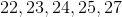Then, subtract the smallest number from the largest number: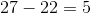Answer:### Example Question #53 : Data Analysis And Probability

Find the range for this set of numbers: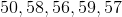Possible Answers:Correct answer:Explanation:

First, order the numbers from least to greatest: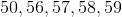Then, subtract the smallest number from the largest: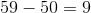Answer:### Example Question #54 : Data Analysis And Probability

Find the range for this set of numbers: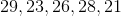Possible Answers:Correct answer:Explanation:

First order the numbers from least to greatest: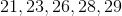Then, subtract the smallest number from the largest: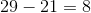Answer:### Example Question #56 : Data Analysis

Find the range in this set of numbers: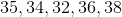Possible Answers:Correct answer:Explanation:

First, order the numbers from least to greatest: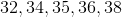Then, subtract the smallest number from the largest number: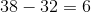Answer:### Example Question #55 : Data Analysis And Probability

Find the range for this set of numbers: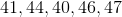Possible Answers:Correct answer:Explanation:

First order the numbers from least to greatest: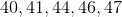Then subtract the smallest number from the largest number: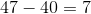Answer:### Example Question #361 : Isee Middle Level (Grades 7 8) Mathematics Achievement

Find the range of this set of numbers: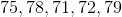Possible Answers:Correct answer:Explanation:

The range is the difference between the smallest and largest elements.

To find the range, first order the numbers from least to greatest: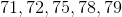Then subtract the smallest number from the largest number: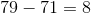Answer:### Example Question #59 : Data Analysis

Find the range of this set of numbers: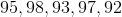Possible Answers: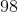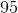Correct answer:Explanation:

First, order the numbers from least to greatest: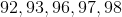Then, subtract the smallest number from the largest number: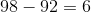Answer:### Example Question #51 : Data Analysis

Find the range of this set of numbers: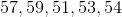Possible Answers: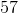Correct answer:Explanation:

First order the numbers from least to greatest: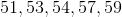Then subtract the smallest number from the largest number: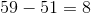Answer:### All ISEE Middle Level Math Resources